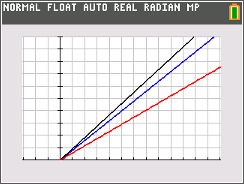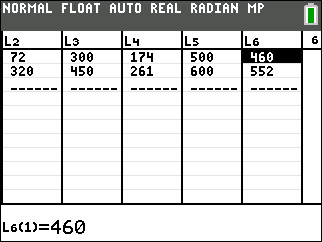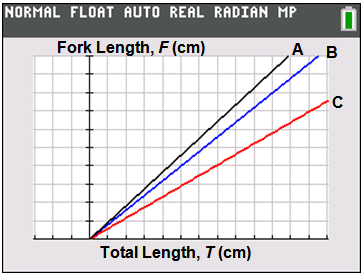••• ##### Device
• TI-84 Plus
• TI-84 Plus Silver Edition
•TI-84 Plus C Silver Edition
•TI-84 Plus CE

# Middle Grades Math: Shark Frenzy

by Texas Instruments#### Overview

Students examine equations in the family of linear functions which are of the form y = m x, each of which correspond to a different family of sharks. They relate the slope to the ratio of the shark’s fork length to its total length. When comparing two sharks of the same length, students conclude that slopes of the lines of the three families decrease with increasing length of the upper caudal lobe

#### Key Steps

•Students will find the slope of a line given two points and interpret the real world meaning of the slope of a linear function in the context of a problem.

•Students will write an equation in the form y = m x to represent a linear relationship between two quantities and relate the value of the slope to the steepness of the graph of the line.